# 25. Rigid Body Moving Freely

Michael Fowler

## Angular Momentum and Angular Velocity

In contrast to angular velocity, the angular momentum of a body depends on the point with respect to which it is defined. For now, we take it (following Landau, of course) as relative to the center of mass, but we denote it by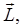following modern usage. This "intrinsic" angular momentum is like the Earth's angular momentum from its diurnal rotation, as distinct from its orbital angular momentum in going around the Sun.

That is,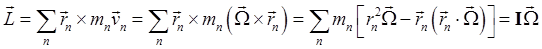whereis the inertia tensor: this just means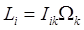.

Explicitly, taking the principal axes as the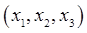axes,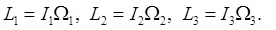For anything with spherical inertial symmetry (such as a cube or a tetrahedron!)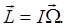.

Landau defines a rotator as a collection of massive particles all on a line. (I guess that includes diatomic molecules, and, for example, CO2, neglecting electrons and nuclear size) We know there are only two physical rotational degrees of freedom for these molecular rotators (thanks to quantum mechanics) and obviously the two principal axes are perpendicular to the line of masses, and degenerate. Again, then,.

## Precession of a Symmetrical Top

A more interesting case is the free rotation (zero external torque) of a symmetrical top, meaningWe can take any pair of orthogonal axes, perpendicular to the body's symmetry axis, as the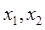axes. We'll choose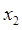, following Landau, as perpendicular to the plane containing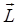and the momentary position of theaxis, so in the diagram hereis perpendicularly out from the paper/screen, towards the viewer.

This means the angular momentum component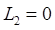and therefore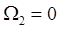. Hence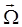is in the same plane as, and so the velocity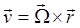of every point on the axis of the top is perpendicular to this plane (into the paper/screen). The axis of the topmust be rotating uniformly about the direction of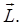The spin rate of the top around its own axis is.

The angular velocity vectorcan be written as a sum of two components, one along the body's axisand one parallel to the angular momentum(these components are shown dashed in the figure)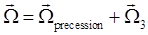The component along the body's axisdoes not contribute to the precession, which all comes from the component along the (fixed in space) angular momentum vector.

The speed of precession follows from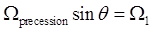, and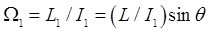, soNote also the ratio of precession rate to spin-around-axis is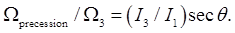This means the precession rate and the spin are very comparable, except when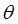is near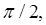when the precession becomes much faster. Remember this is the body's precession with no external torque, and is clearly completely different -- much faster precession -- than the familiar case of a fast spinning top under gravity.

### Throwing a Football...

If you throw a football and manage to give it only spin about the long axis, it will stay pointing that way (apart from drag effects, which will tend to line up spin direction with velocity). If when you throw it you also add some angular velocity along a shorter axis, it will precess (wobble). Given the angle, the ratio of precession to spin is fixed.

If you take a ball that is already spinning fast about its long axis, have the two ends of the long axis (its points) in your palms, then, as you throw it, give it a quick twist by moving one hand downwards and the other up as you throw, to give it significant angular velocity about a short axis, at the same time keeping the fast spin about the long axis, once the ball leaves your hands, the angle between the spin and the total angular momentum, the angle of wobble, is completely determined by the ratio of the two angular velocities.

## Equations of Motion for Rigid Body with External Forces

### Translation

A free rigid body has six degrees of freedom (for instance, the coordinates of the center of mass and the orientation of the body). Therefore, there are six equations of motion, three for the rate of change of spatial position of the center of mass, in other words for the components of the velocity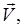and three for the rate of change of orientation, the angular velocity.

These equations are of course nothing but Newton's laws, easily derived by summing over the set of equations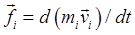for each particle. Denoting the total momentum of the body by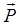,and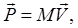where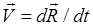is the velocity of the center of mass. (This can be established by differentiating with respect to time the definition of the center of mass,)

The total force on all the particles is a sum of the total external force on the body and the sum of internal forces between particles -- but these internal forces come in equal and opposite pairs, from Newton's Third Law, and therefore add to zero.

The bottom line, then, is that the rate of change of momentum of a rigid body equals the total external force on the body. If this force is from a time-independent potential, thenbecause if the body is moved through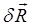(without rotation, hence the partial derivative), each individual particle moves through the same, the work done by the external potential on theparticle is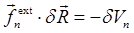, and summing over all the particles givesgiving the above equation as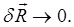### Rotation

To derive the equation of motion for rotation of a rigid body, we choose the inertial frame in which the center of mass is momentarily at rest, and take the center of mass as the origin.

The rate of change of angular momentum about the center of mass (origin),where we dropped theterm because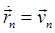is parallel to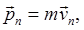then we used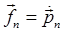to get the total moment of the external forces about the center of mass, the torque.

The angular momentum about the center of mass is the same in any inertial frame, since the extra term on adding a velocity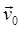to each mass is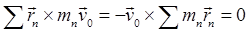from the definition of the center of mass.

If the center of mass is not at the origin, denote the particle coordinates by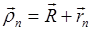in the usual notation, so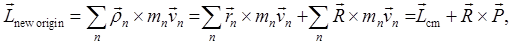a sum of an intrinsic ("spin") angular momentum and an extrinsic ("orbital")angular momentum.

Similarly, if the torque of external forces relative to the center of mass is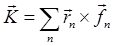as defined above, then relative to the new origin the torque is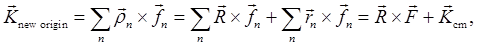that is, the torque about the new origin is the torque about the center of mass plus the torque about the new origin of the total external force acting at the center of mass.

An important special case is that of a couple: a pair of equal but oppositely directed forces, acting along parallel but separated lines (like two hands oppositely placed turning a steering wheel). The forces add to zero, so from the above equation a couple exerts the same torque about any origin.

More generally, the term couple is often used (including by Landau) to refer to any set of forces that add to zero, but give a nonzero torque because of their lines of action, and such a set give the same torque about any origin.

Exercise: prove that for a rigid body freely falling in a uniform gravitational field, the angular momentum about the center of mass remains constant, but about another point it will in general be changing. What about a charged rigid body moving in space (no gravity) through a uniform electric field?